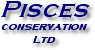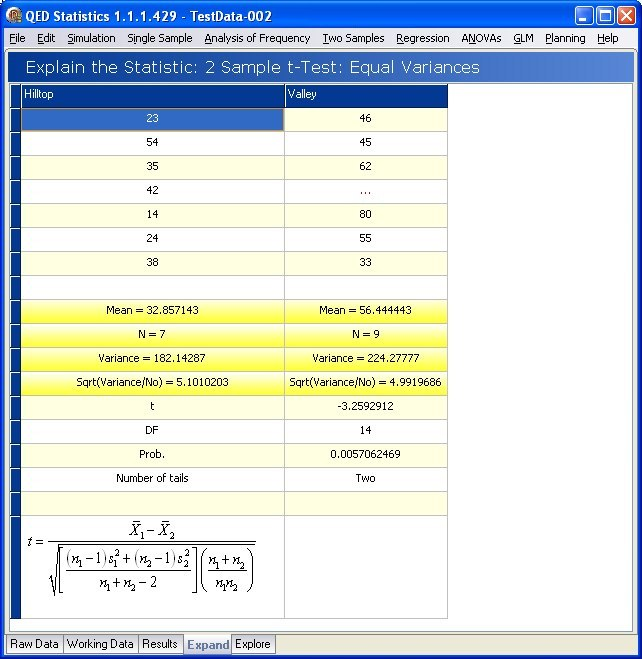Two Sample tests: mean, variance and frequency comparisons - in an instant

The Two Samples menu gives 8 methods for comparing two-sample data:

• t-Test - Equal Variance - Balanced to compare two means when both samples are assumed to have the same variance and both samples have the same number of observations.
• t-Test - Equal Variance - Unbalanced to compare two means when both samples are assumed to have the same variance and the samples have different numbers of observations.
• t-Test - Unequal Variance - If the samples cannot be assumed to have the same variances.
• Mann Whitney - a non-parametric test to determine if there is a significant difference between the medians of two samples.
• Compare Variance (F Test) - to test if the variances of two samples are the same.
• Chi-squared - to test two sets of frequencies for a significant difference.
• Paired t-Test - to compare the means of paired samples.
• Wilcoxon Matched Pairs (Signed rank) - the non-parametric test for difference between matched pairs.Simple intuitive setup dialogs mean you can carry out a
two-sample analysis in seconds.

When the analysis is done, Expand & Explore take you step-by-step
through the entire calculation.